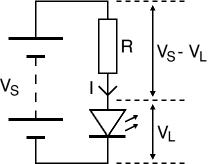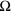# Calculating LEDs resistor valueLEDs must have a resistor connected in series to limit the current flowing through them, otherwise they will burn out almost instantly.

The resistor value, R is given by:

 R = (VS - VL) / I

VS
= the supply voltage
VL = the LED voltage (usually 2V, but may also be 4V for blue and white LEDs)
I = the LED maximumcurrent (e.g. 10mA = 0.01A, or 20mA = 0.02A)

Make sure the LED current you choose is less than the maximum permitted and then convert
the current to amps (A)
so the calculation will give the resistor value in ohms. To convert
mA to A divide the current in mA by 1000 because 1mA = 0.001A.

If the calculated value is not available choose the nearest standard resistor value which is
greater, so that the current will be a little less than you chose. In fact you may wish to choose
a greater resistor value to reduce the current (to increase battery life for example) but this will
make the LED less bright.

#### For example

Assuming the supply voltage is  VS = 9V , and you have a red LED ( VL = 2V ), requiring a current
I = 20mA = 0.020A, R = (9V - 2V) / 0.02A = 350, so choose a 390resistor (the nearest
standard value which is greater).